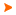Podaci o VU Početna stranicaArhiva nastavnih programaArhiva ak. kalendaraDynamics of Multibody Systems
 Abbreviation: DMS_ENG Load: 30(L) + 0(E) + 15(LE) + 0(CE) + 0(PEE) + 0(FE) + 0(S) + 0(DE) + 0(P) + 0(FLE) + 0()
 Lecturers in charge: prof. dr. sc. Zdravko Terze Lecturers: doc. dr. sc. Dario Zlatar ( Lectures ) Viktor Pandža mag. ing. mech. ( Laboratory exercises ) Course description: Course objectives: To describe methods, algorithms and application of dynamical and mathematical modelling and computational preparation of dynamical models with the aim of numerical analysis and dynamic simulation of engineering systems with complex kinematical structure and spatial (3D) motion such as robots and manipulators, vehicles, windturbines, flight vehicles (with fixed and rotary wings), biomechanical systems and others.Enrolment requirements and required entry competences for the course: Mechanics II (Solids Kinematics and Dynamics)Student responsibilities: Students are required to regularly and actively participate in class and to accomplish the assigned tasks. The examination consists of written and oral parts. The final score is obtained as a result of written and oral exames.Grading and evaluation of student work over the course of instruction and at a final exam: Activities that are valued: written exam 50% oral exam 50%Methods of monitoring quality that ensure acquisition of exit competences: Continuity of students" work will be monitored through activities and exercises in the classroom and through individual and team execution of tasks. Acquired knowledge of students will be monitored through discussions during lectures and exercises. If necessary, the initial level of competence of students will be checked. The quality and success of the course will be monitored through the evaluation at the end of the semester, based on the success of the students at the final exam. Continuous selfevaluation will be done through the comparison of the instructional content of the course with the similar courses at EU universities. Also, the contacts with the professors at EU universities will be organized at regular basis, discussing relevant academic issues.Upon successful completion of the course, students will be able to (learning outcomes): analyze and apply mathematical models to calculate dynamic response of engineering systems with complex kinematical structure and threedimensional domain of motion derive and use mathematical equations for definition of the kinematical constraints of lower and higher kinematic pairs derive and apply mathematical equations of skleronomic and rheonomic kinematical constraints use and calculate derived formulations during design of computational models for dynamic analysis and numerical simulation of multibody systems dynamicsLectures 1. Introduction. Computational dynamics of structural systems: numerical casestudies in mechanical engineering. Elements and structure of mechanical models.2. Dynamics of rigid bodies: basic equations and princples, NewtonEuler equations. Dynamic equations of "free" rigid bodies systems.3. Kinematical constraints, characteristics and principles: scleronomic and rheonomic kinematical constraints, unilateral contacts. Mathematical modelling of kinematical constraints.4. Kinematical constraint matrix: mathematical structure and characteristics. Kinematical synthesis of mechanical system.5. Numerical methods of kinematical synthesis.6. Dynamic equations of constrained multibody systems. Minimal and descriptor form of mathematical model.7. Computational characteristics of different forms of mathematical model. Numerical methods of reduction of dimensionality of system dynamical equations.8. Orthogonalcomplement matrix: numerical methods, characteristics and utilization principles.9. Dynamic simulation of multibody structural systems. Preparation of mathematical models for dynamic simulation algorithms.10. Numerical methods: ODE and DAE systems of differential equations, characteristics and basic principles of timeintegration.11. ODE and DAE systems timeintegration schemes.12. Constraint violation stabilization algorithms. Computational treatment of numerically stiff problems.13. Computational methods for solving problems of inverse dynamics.14. Dynamic simulation of systems with variable kinematic structure.15. Presentation of different casestudies in computational dynamics of structural systems.Exercises 1. Dynamic analysis and simulation of structural systems: examples of application cases.2. Basic architecture of computational procedures and program packages: introduction.3. Basic architecture of computational procedures and program packages: continuation.4. Numerical casestudy assignment: dynamic simulation of structural.5. Mechanical model synthesis for assigned numerical case: objectives, assumptions, elements of discretization.6. Definition of kinematical structure of the system. Mathematical modelling of kinematical constraints: introduction.7. Mathematical modelling of kinematical constraints: continuation.8. Numerical procedures for the model kinematical synthesis: introduction.9. Numerical procedures for the model kinematical synthesis: continuation. Redundant kinematical constraints.10. Computational synthesis of mathematical model of dynamics of the system: introduction.11. Computational synthesis of mathematical model of dynamics of the system: introduction.12. Model preparation for the system dynamic simulation.13. Numerical integration of differential equations of system dynamics: introduction. A choice of numerical method for timeintegration. Definition of parameters of integration.14. Numerical integration of differential equations of system dynamics: continuation. Constraint violation proofprocedure. Results of dynamic simulation.15. Dynamic simulation with different parameters of integration. Interpretation of the results. Disscussion.
 Lecture languages: en
 Compulsory literature: 1. Terze, Z.: Eiber, A. (University of Stuttgart), Introduction to Dynamics of Multibody Systems, FSB Zagreb, e-book, 2002. 2. Bauchau, O.: Flexible Multibody Dynamics, Willey, 2011.
 Recommended literature: 3. Eich-Soellner, E.; Fuehrer, C.: Numerical Methods in Multibody Dynamics, B. G. Teub. Stuttgart, 1998.Legend L - Lectures FLE - Practical foreign language exercises - E - Exercises LE - Laboratory exercises CE - Project laboratory PEE - Physical education excercises FE - Field exercises S - Seminar DE - Design exercises P - Practicum * - Not graded| Početna stranica | Visoka učilišta | Korisničke stranice | | Na vrh | Copyright (c) 2006. Ministarstva znanosti, obrazovanja i športa. Sva prava zadržana. Programska podrška (c) 2006. Fakultet elektrotehnike i računarstva. Oblikovanje(c) 2006. Listopad Web Studio. Posljednja izmjena 2019-06-07